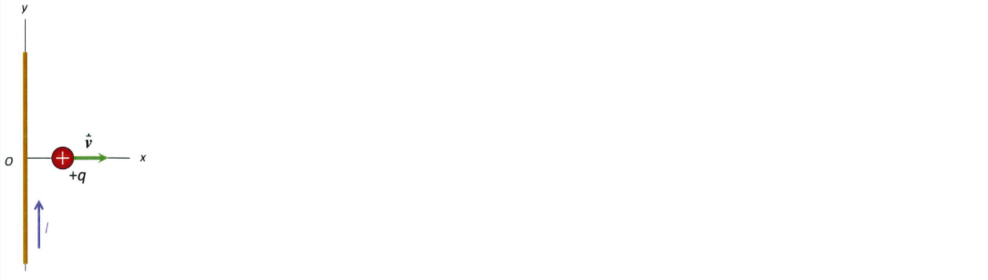# Problem: A long, straight wire lies along the y-axis and carries current in the positive y-direction. A positive point charge moves along the x-axis in the positive x-direction. The magnetic force that the wire exerts on the point charge is inA. the positive x-direction.B. the negative x-direction.C. the positive y-direction.D. the negative y-direction.E. none of the above.

90% (67 ratings)
###### Problem Details

A long, straight wire lies along the y-axis and carries current in the positive y-direction. A positive point charge moves along the x-axis in the positive x-direction. The magnetic force that the wire exerts on the point charge is in

A. the positive x-direction.

B. the negative x-direction.

C. the positive y-direction.

D. the negative y-direction.

E. none of the above.